# 如何在 powerbi 中使用 dax 返回某一值对应的字段名

## 原始数据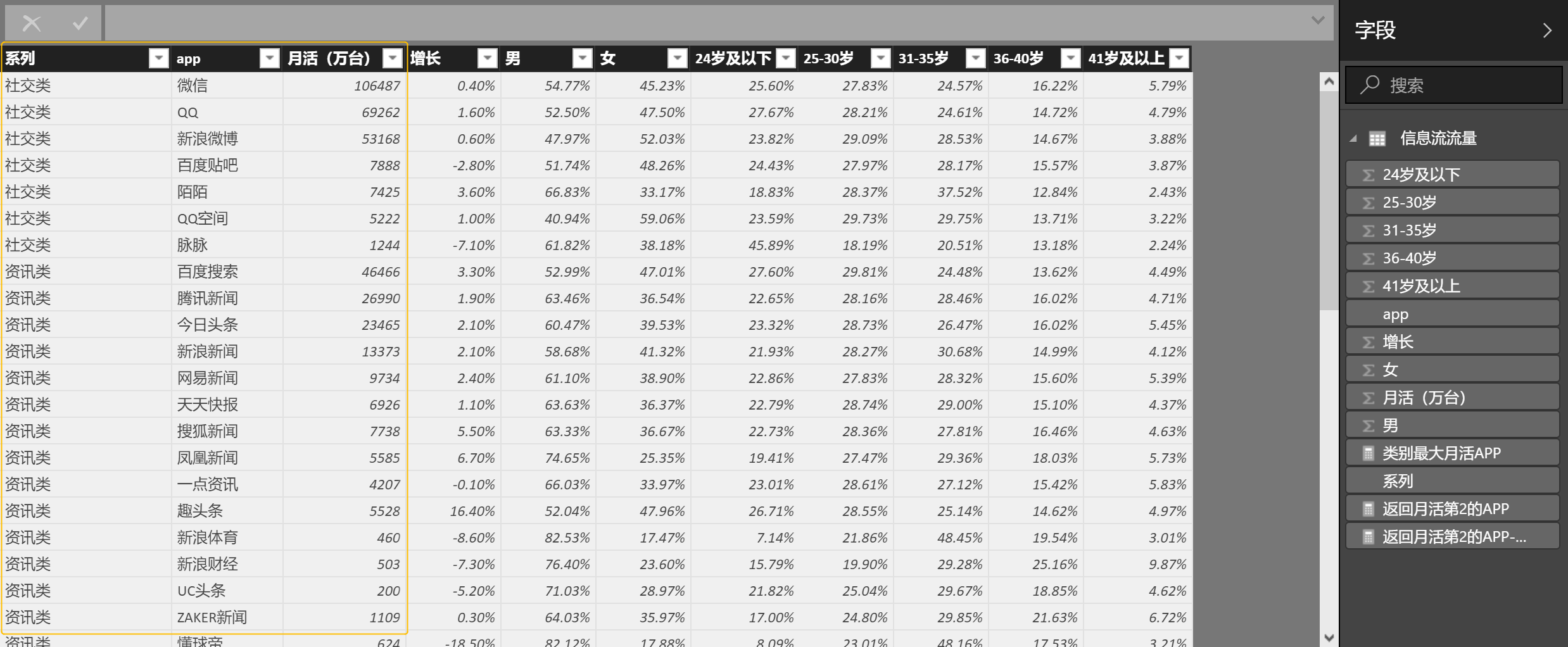## 需要解决的问题一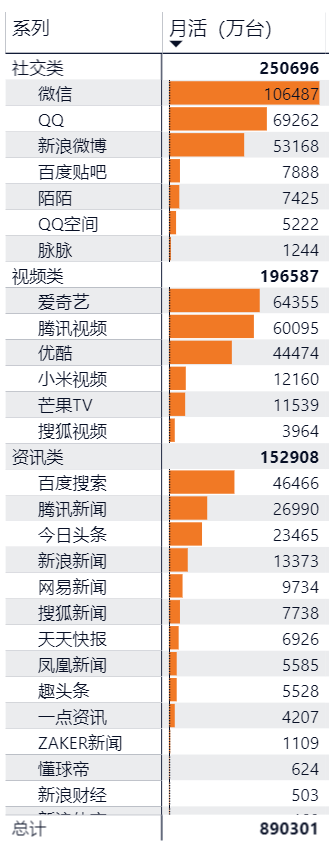### 解决思路：

``类别最大月活APP = CALCULATE(MAX('信息流流量'[app]),FILTER('信息流流量','信息流流量'[月活（万台）]=MAX('信息流流量'[月活（万台）])))``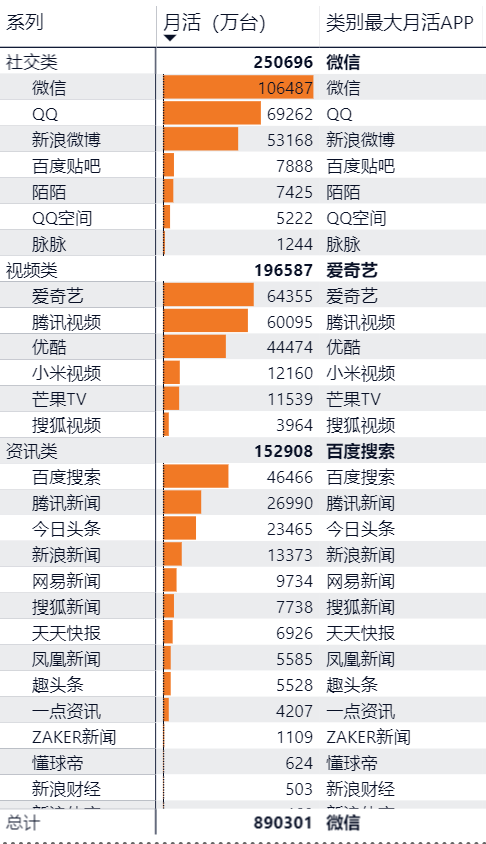``类别最大月活APP = IF(HASONEVALUE('信息流流量'[app]),"", CALCULATE(MAX('信息流流量'[app]),FILTER('信息流流量','信息流流量'[月活（万台）]=MAX('信息流流量'[月活（万台）]))))``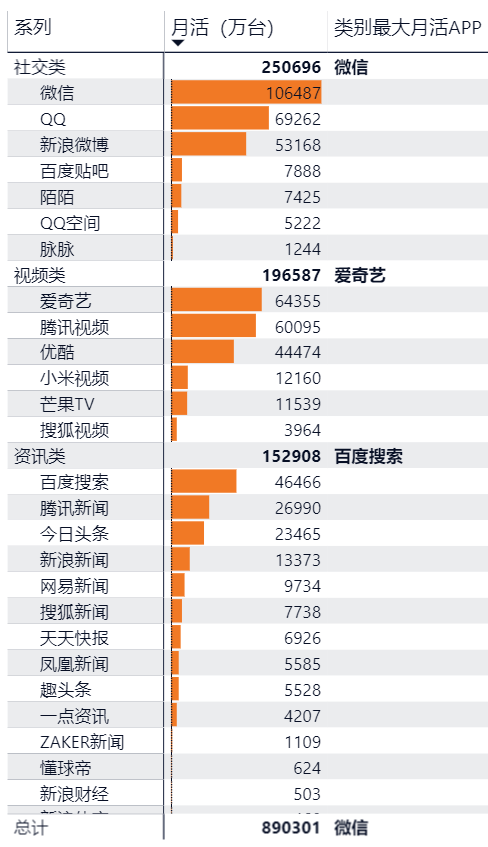## 那如果我们需要返回月活排名第二，或者第三的app呢？

### 思考

`系列内APP排名 = RANKX(ALLEXCEPT('信息流流量','信息流流量'[系列]),CALCULATE(SUM([月活（万台）])))`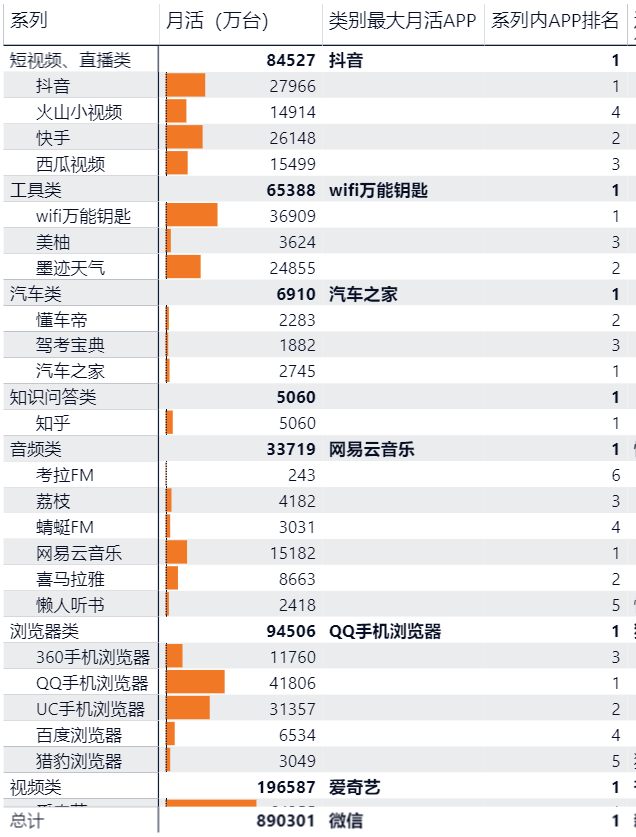### 使用前面的思路我们套入公式

``返回月活第2的APP = CALCULATE(MIN('信息流流量'[app]),FILTER(VALUES('信息流流量'[app]),RANKX(ALLEXCEPT('信息流流量','信息流流量'[系列]),CALCULATE(SUM([月活（万台）])))=2))``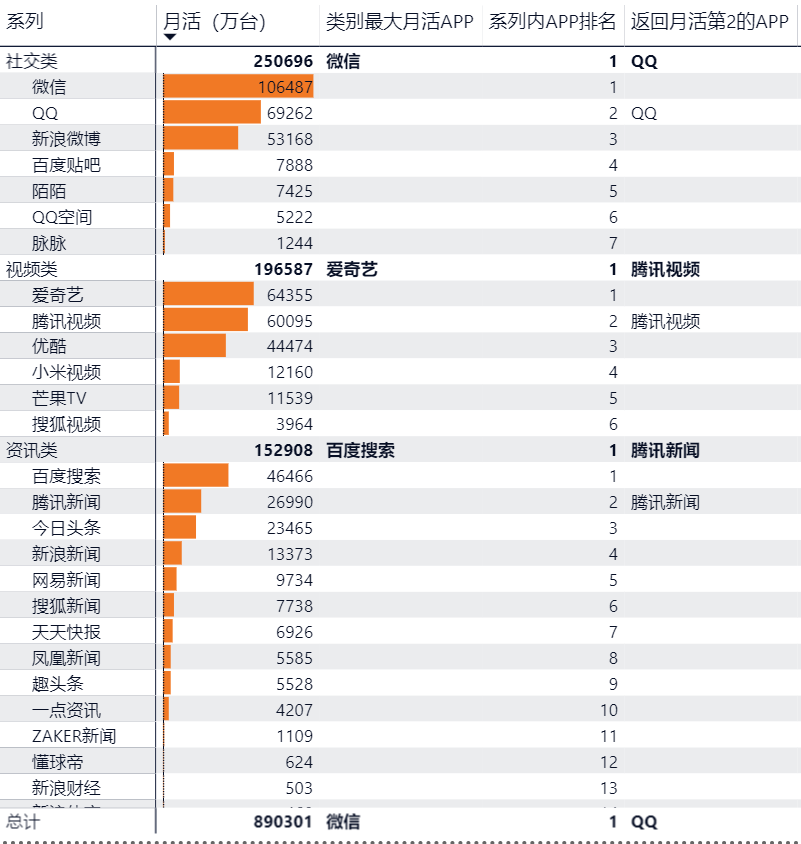### 可以将公式调整为VAR更好理解

``````返回月活第2的APP-VAR =
VAR n = 2
VAR app_list = VALUES('信息流流量'[app])
VAR app_filter = FILTER(app_list,RANKX(ALLEXCEPT('信息流流量','信息流流量'[系列]),CALCULATE(SUM('信息流流量'[月活（万台）])))=n)
RETURN CALCULATE(MIN('信息流流量'[app]),app_filter)``````